# ShuffleNet-V1 Trained onImageNet Competition Data

Identify the main object in an image

Released in 2017, ShuffleNet is designed specially for mobile devices with very limited computing power. Its architecture utilizes two new operations: pointwise group convolutions and channel shuffling, greatly reducing computational cost while maintaining accuracy.

Number of layers: 203 | Parameter count: 1,420,152 | Trained size: 7 MB |

## Performance

• This model achieves a top-1 accuracy of 67.4% on the original ImageNet validation set.

## Examples

### Resource retrieval

Get the pre-trained net:

 In:=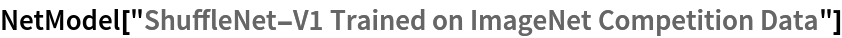Out=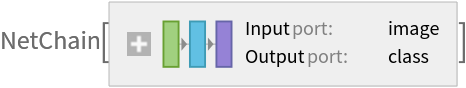### Basic usage

Classify an image:

 In:=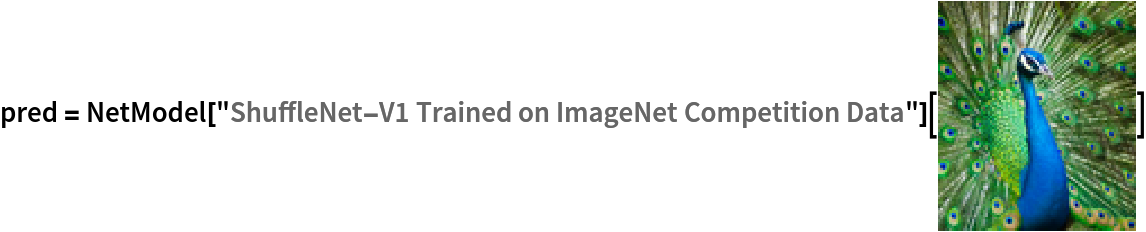Out=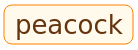The prediction is an Entity object, which can be queried:

 In:=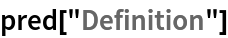Out=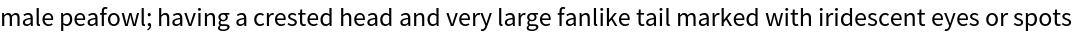Get a list of available properties of the predicted Entity:

 In:=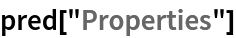Out=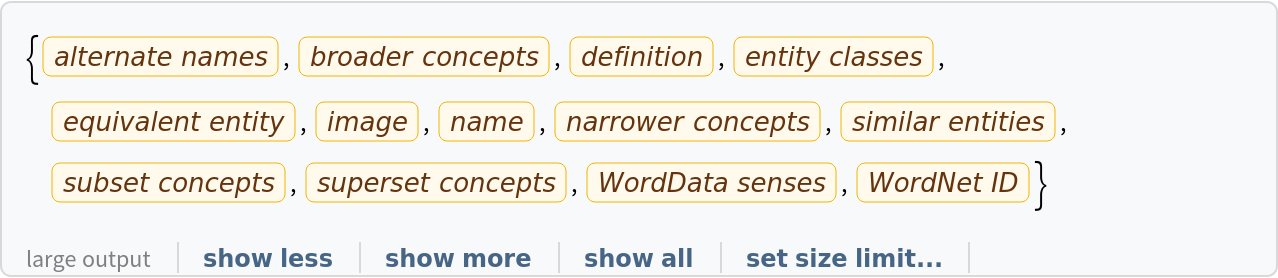Obtain the probabilities of the 10 most likely entities predicted by the net:

 In:=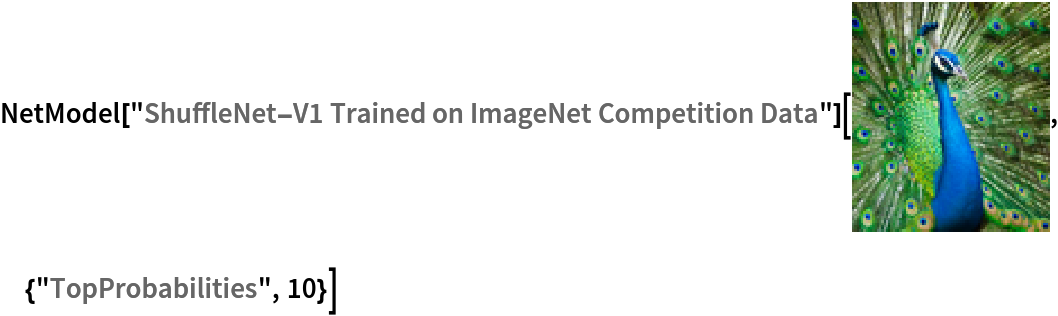Out=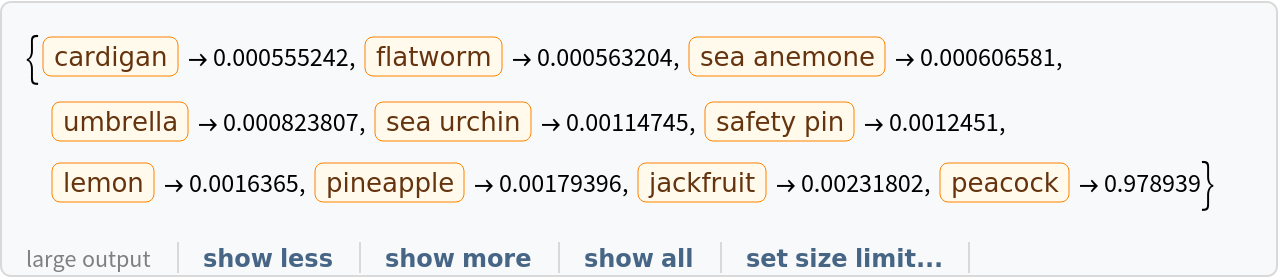An object outside the list of the ImageNet classes will be misidentified:

 In:=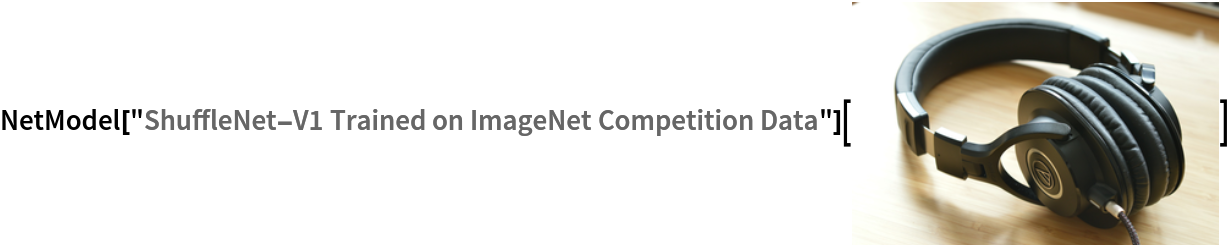Out=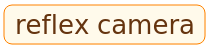Obtain the list of names of all available classes:

 In:=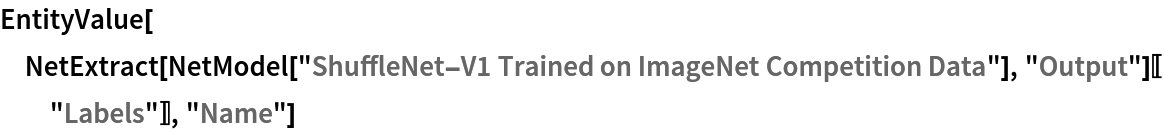Out=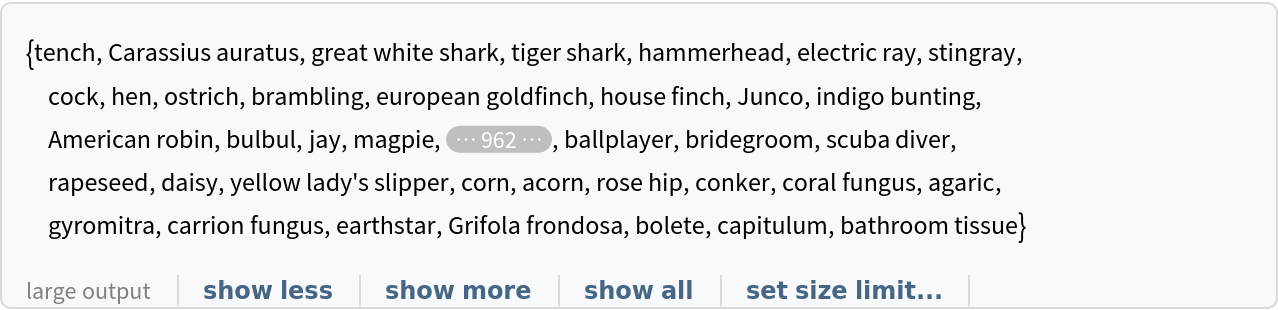### Feature extraction

Remove the last four layers of the trained net so that the net produces a vector representation of an image:

 In:=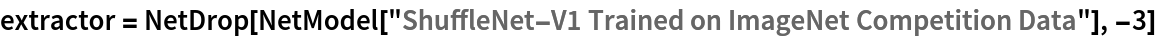Out=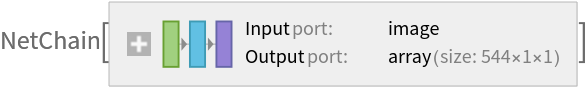Get a set of images:

 In:=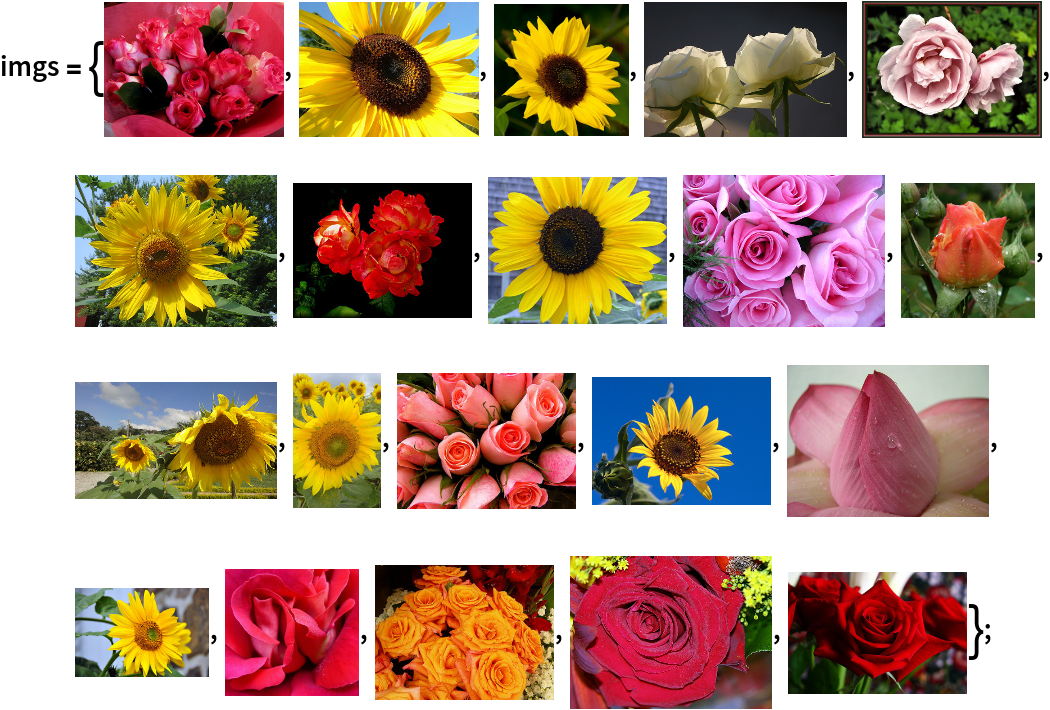Visualize the features of a set of images:

 In:=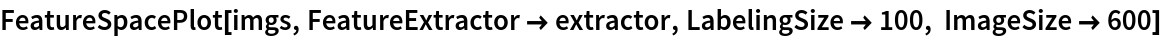Out=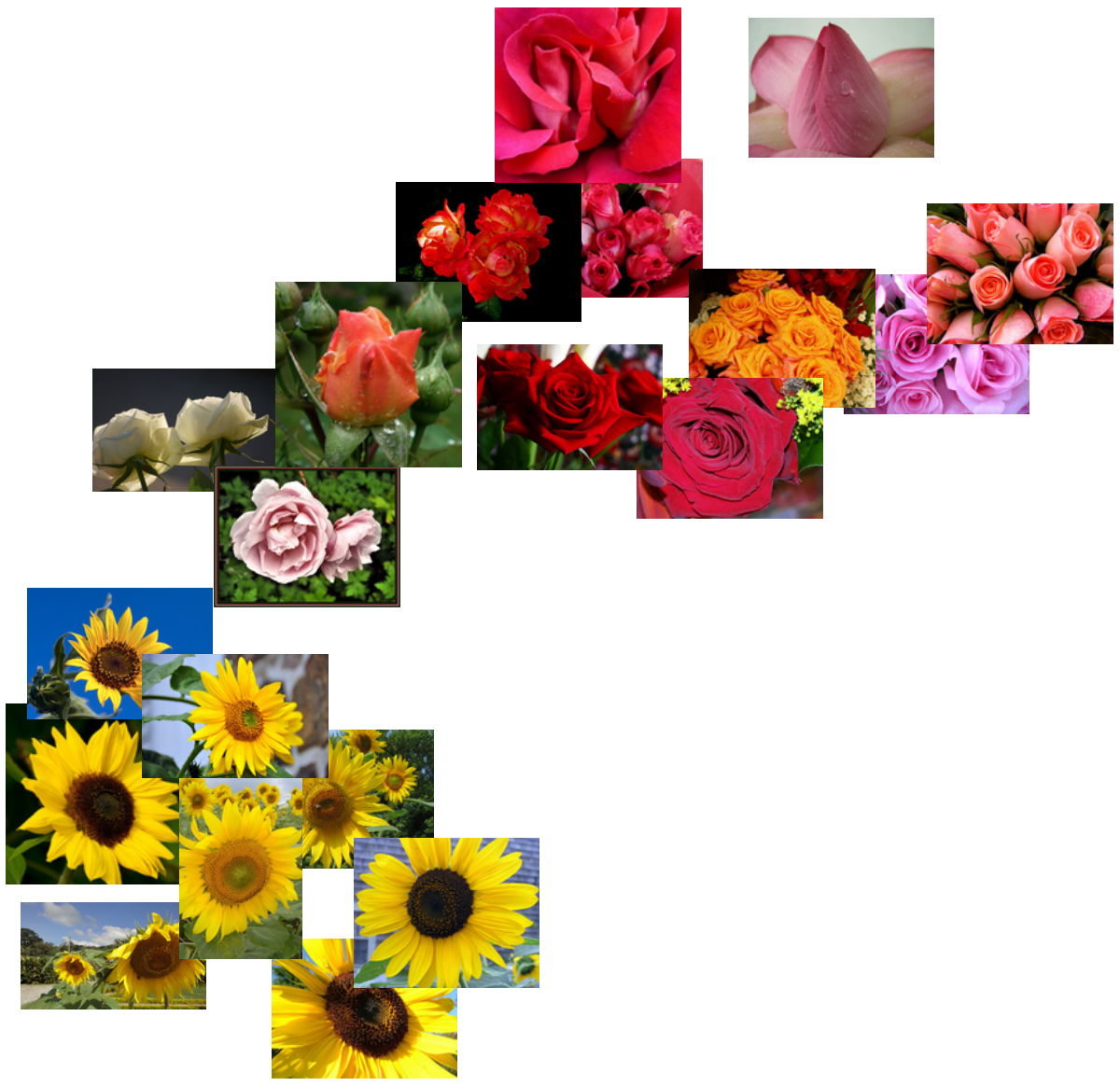### Visualize convolutional weights

Extract the weights of the first convolutional layer in the trained net:

 In:=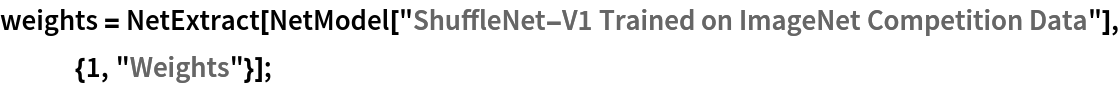Show the dimensions of the weights:

 In:=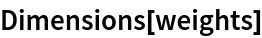Out=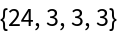Visualize the weights as a list of 24 images of size 3⨯3:

 In:=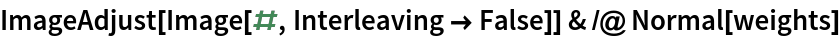Out=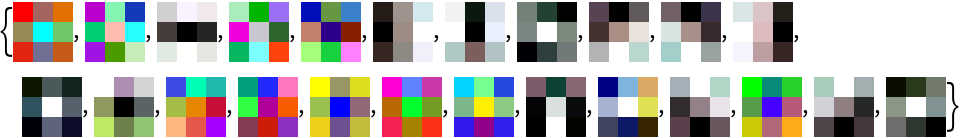### Transfer learning

Use the pre-trained model to build a classifier for telling apart images of sunflowers and roses. Create a test set and a training set:

 In:=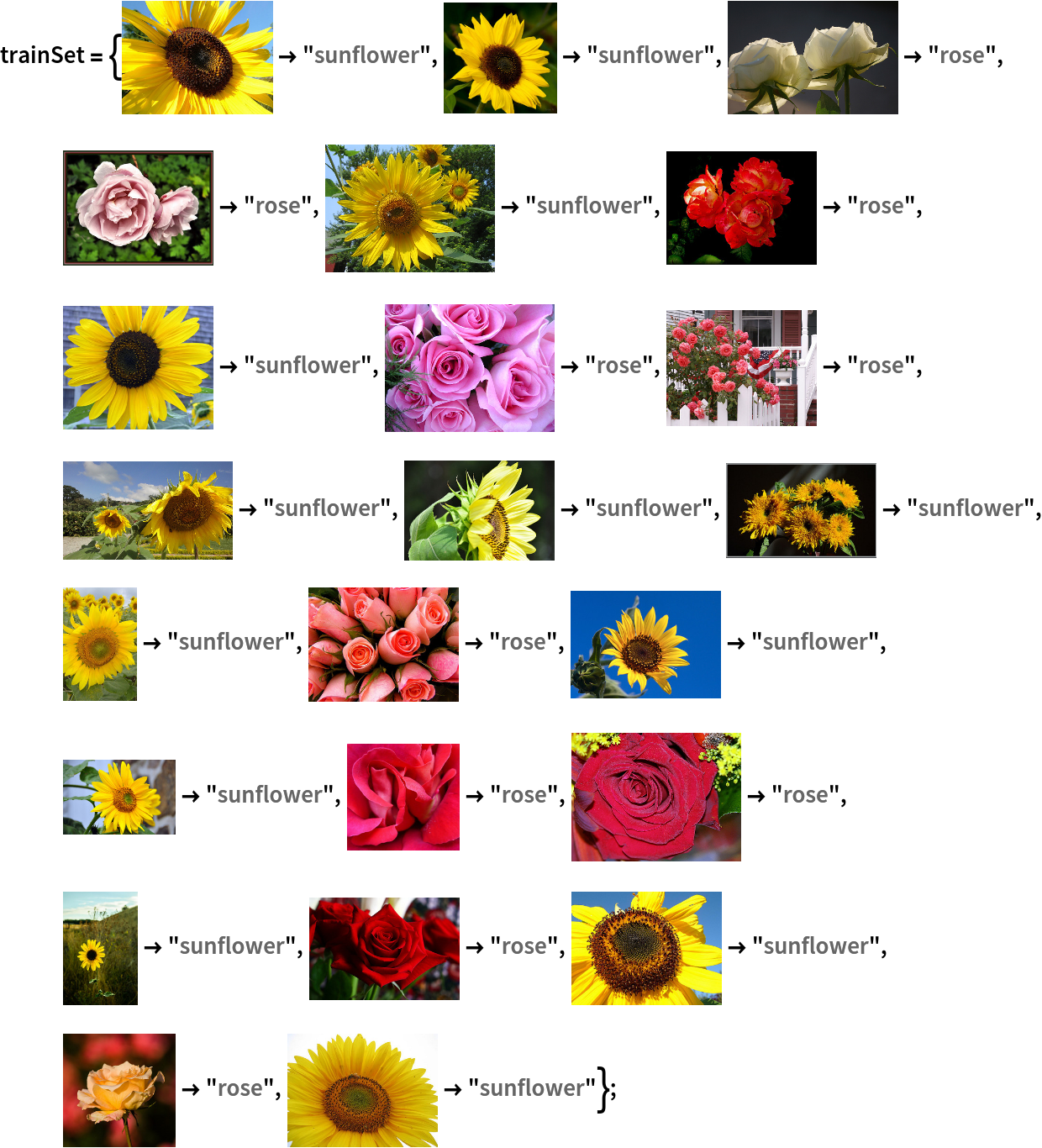In:=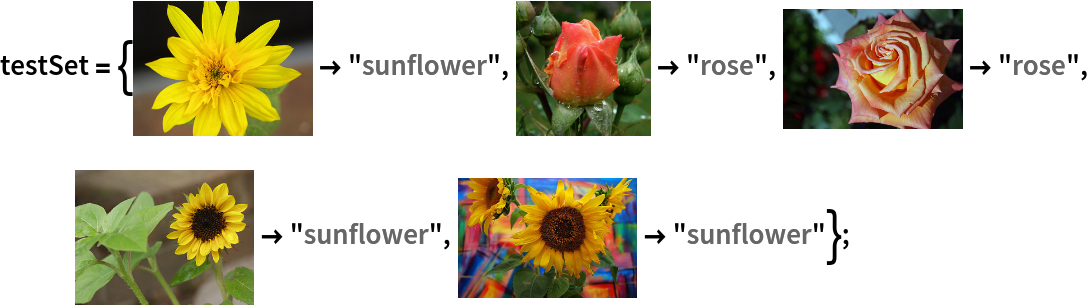Remove the last layers from the pre-trained net:

 In:=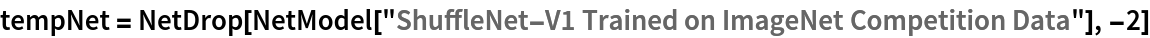Out=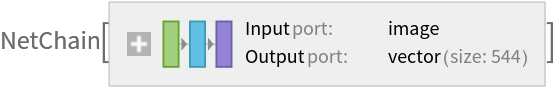Create a new net composed of the pre-trained net followed by a linear layer and a softmax layer:

 In:=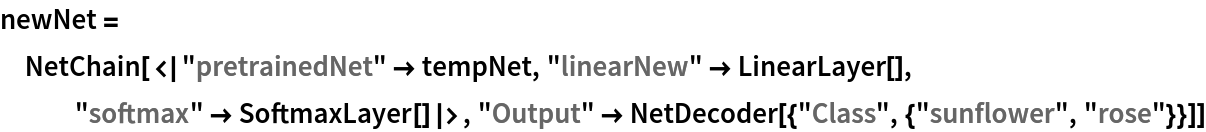Out=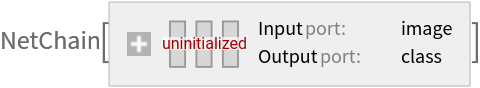Train on the dataset, freezing all the weights except for those in the "linearNew" layer (use TargetDevice -> "GPU" for training on a GPU):

 In:=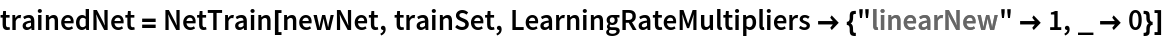Out=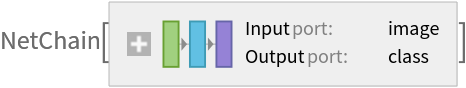Accuracy obtained on the test set:

 In:=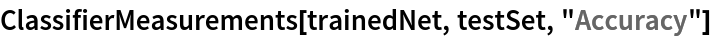Out=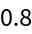### Net information

Inspect the number of parameters of all arrays in the net:

 In:=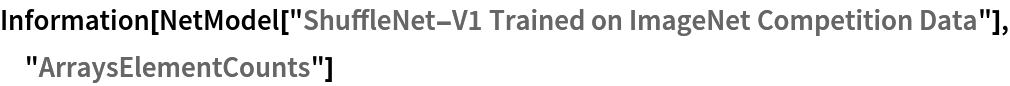Out=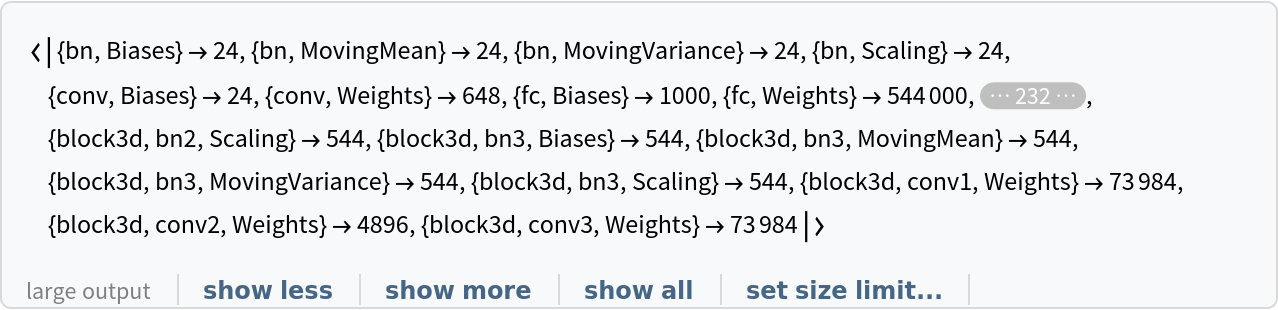Obtain the total number of parameters:

 In:=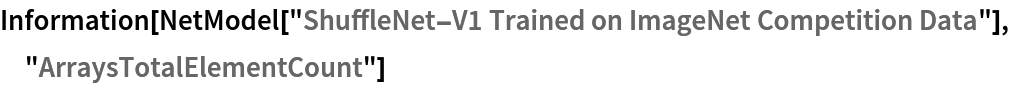Out=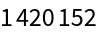Obtain the layer type counts:

 In:=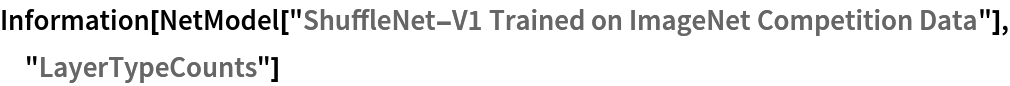Out=Display the summary graphic:

 In:=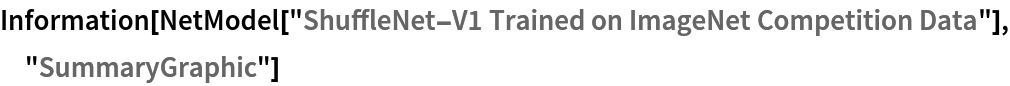Out=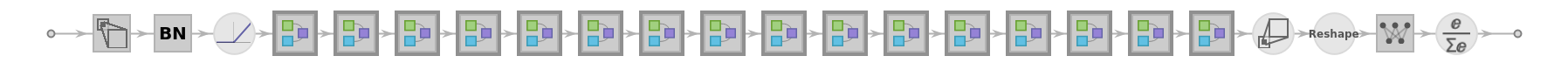### Export to ONNX

Export the net to the ONNX format:

 In:=Out=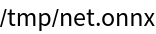Get the size of the ONNX file:

 In:=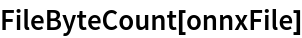Out=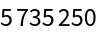The size is similar to the byte count of the resource object:

 In:=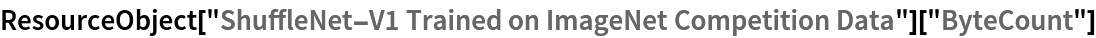Out=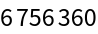Check some metadata of the ONNX model:

 In:=Out=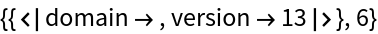Import the model back into the Wolfram Language. However, the NetEncoder and NetDecoder will be absent because they are not supported by ONNX:

 In:=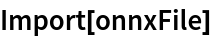Out=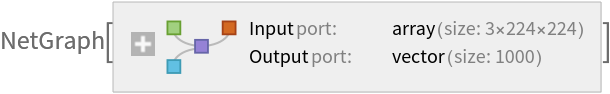## Reference

• X. Zhang, X. Zhou, M. Lin, J. Sun, "ShuffleNet: An Extremely Efficient Convolutional Neural Network for Mobile Devices," arXiv:1707.01083v2 (2017)
• Rights: BSD 3-Clause